# DESIGN OF STEEL COMPRESSION MEMBERS

A structural member loaded axially in compression is generally called a compression member. Vertical compression members in buildings are called columns, posts or stanchions. A compression member in roof trusses is called struts and in a crane is called a boom.

Columns which are short are subjected to crushing and behave like members under pure compression. Columns which are long tend to buckle out of the plane of the load axis.

#### THEORY OF COLUMNS

Euler’s formula for critical load for a pin-ended column subjected to axial load is

Where, L = length of column between the hinged ends,

E = modulus of elasticity, and

I = moment of inertia of the column section.

The column will become unserviceable if the loads are larger than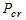. In the Euler equation, it is assumed that stress is proportional to strain, therefore,

Critical Stress =

Where, A= area of cross-section, and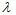= slenderness ratio

#### VARIOUS END CONDITIONS

Columns with length L and effective length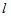are shown in figure below:

#### Strength of an Axially Loaded Compression Members

Maximum axial compression load permitted on a compression member,

Where, P = axial compressive load (N),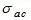= permissible stress in axial compression (MPa)

A = effective cross-sectional area of the member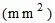Indian Standard IS 800: 1984

It stipulates that the direct stress on the cross-sectional area of axially loaded compression members should not exceed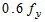nor the permissible stress calculated using Merchant – Rankine formula.

Permissible stress in axial compression (MPa):

Where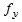= yield stress of steel in MPa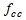= elastic critical stress in compression =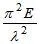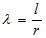= slenderness ratio of the member

Where,= effective length of the member

r = appropriate radius of gyration of the member

E = modulus of elasticity = 200000 MPa, and

n = a factor assumed as 1.4

EFFECTIVE LENGTH OF COMPRESSION MEMBER

Table below gives the values of effective length recommended by the Indian Standard, IS 800. The actual length L of the compression member should be taken as the length from centre-to-centre of intersection of supporting members or the cantilevered length in the case of free standing struts.

Table: Equivalent length for various end conditions

Note:

1. L is the unsupported length of compression member.
2. For battened struts, the effective length should be increased by 10%.